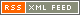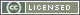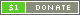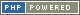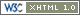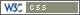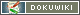# Comparison Operators

Comparison Operators compare two terms and return a Boolean.

Symbol Type Name Description Example
Equality Comparison
Standard comparison
== Infix Equal to `true` if both terms are equal ` a == b `
!= Infix Not equal to `true` if both terms are not equal ` a != b `
<> Infix Not equal to `true` if both terms are not equal ` a <> b `
=== Infix Identical to `true` if both terms are identical (equal, and of the same type) ` a === b `
!== Infix Not identical to `true` if both terms are not identical (are not equal, or are of different types) ` a !== b `
String comparison
eq Infix Equal to `true` if both terms are equal ` a eq b `
ne Infix Not equal to `true` if both terms are not equal ` a ne b `
Type checking
is Infix Is `type` `true` if the left term is of the type specified by the right term ` a is int `
Relational Comparison
Standard comparison
< Infix Less than `true` if the left term is less than the right term ` a < b `
<= Infix Less than or equal to `true` if the left term is less than or equal to the right term ` a <= b `
> Infix Greater than `true` if the left term is greater than the right term ` a > b `
>= Infix Greater than or equal to `true` if the left term is greater than or equal to the right term ` a >= b `
String comparison
lt Infix Less than `true` if the left term is less than the right term ` a lt b `
le Infix Less than or equal to `true` if the left term is less than or equal to the right term ` a le b `
gt Infix Greater than `true` if the left term is greater than the right term ` a gt b `
ge Infix Greater than or equal to `true` if the left term is greater than or equal to the right term ` a ge b `
Presence checking
in Infix Search `true` if the left term is found in the right term ` a in b `

Unlike most other Operators, Comparison Operators cannot be chained. So, whereas the expression `a + b + c` is valid, `a < b < c` will not have the desired effect. Instead, it should be written as `a < b && b < c`.

Two sets of Comparison Operators are provided, with a standard set for normal checking, and an additional set for easy use of string comparison.

### Standard comparison

When using standard comparison, the left and right terms are compared as integer Numbers if at all possible, and as Strings otherwise. For instance, comparing "blue" and "red" would give `false`, but comparing "+10" with "10.0" would give `true`. This behaviour may not always be expected, and is because the standard comparison operators use "lazy" comparison. The solution is to use the string comparison operators, which evaluate terms as strings; or the identicality operators (`===` and `!==`), which do not try to convert value types.

### String comparison

When using the string comparison operators, terms are compared as Strings, according to the comparison directives in place. The string comparison operators are therefore safe for use when lazy comparison is undesirable, or when alternative behaviour is required (such as natural-order comparison, and case-insensitivity, both of which can be achieved by using the `Use` command to change the comparison directives). However, comparison as Strings means that, for instance, `9` will be greater than `10` (just like `"b"` is greater than `"ab"`).

### Alternatives

If necessary, the `compare()` function can be used, as it allows the comparison method to be chosen on a per-call basis.